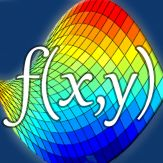Ελληνικά

Προσφέρουμε καθημερινά ΔΩΡΕΑΝ λογισμικό με άδεια χρήσης, που θα αγοράζατε σε διαφορετική περίπτωση!\$1.99
ΕΛΗΞΕ

# iPhone Giveaway of the Day - VisualMATH 4D

VisualMATH 4D is a graphical calculator and lets you draw graphs of your mathematical functions in 2D, 3D and 4D.
\$1.99 ΕΛΗΞΕ

This giveaway offer has been expired. VisualMATH 4D is now available on the regular basis.

VisualMATH 4D is a graphical calculator and lets you draw graphs of your mathematical functions in 2D, 3D and 4D with a time-variable (t).

Features:

- draw graphs with one or two variables like f(x), f(x,y)
- visualize your function in 4 dimensions with a time-variable
- compute value tables
- definite integral and derivative - functions
- matrices, vectors, determinante, inverse, transpose,
- trigonometrical functions like sin, cos, tan, sec
- rounding-functions, floor, ceil, round,
- sum and product-function
- save your functions and make screenshots
- modify your graph-range and set customized color options
- rotate and zoom your graph in three dimensions
- display multiple graphs

The app is easy to use and helps students and engineers to visualize their mathematical functions and it can be used as a professional graphical calculator.

Ronny Weidemann

Education

4.1

16.6 MB

4+

English

### Συμβατότητα: